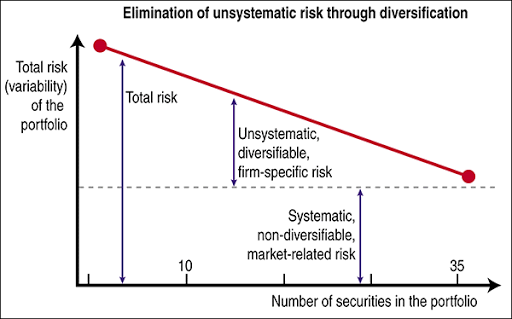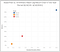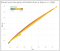# How Much Bitcoin Should You (Reasonably) Own?

## A data-driven how-to guide on quantifying risk for portfolios with crypto exposure using Python, Modern Portfolio Theory, Sharpe Ratio, and Monte Carlo Simulations.A graphical breakdown of Systematic vs Unsystematic risk. Diversification can reduce unsystematic risk. Source

# The Problem — How Does Crypto Affect Your Portfolio Volatility?

Let’s say you’re an average 20-something year old with about \$16,000 in retirement savings. You know a little bit about “investing” and have most of your money in a Vanguard Total Stock Market Index and a Vanguard US Bond Index. You also like to play around with some individual stock picking and crypto currencies on Robinhood, because what if I find the next big crypto before it blows up?? Imagine what would happen if I put in \$1000 today and it goes up by 9150% in one year like ETH? I’d be rich!!

So then you decide to toss about a grand into Robinhood and see what happens. Making money isn’t so hard. After all — you’ve gained 50% in two months on AMC and BB, so why does everybody get excited about trying to make only 7 or 8% in a year?

In this article, we’re going to try to quantify how much risk you’re really taking on with this strategy. Specifically, the questions I’ll try to answer are:

• How risky is (or was, over a specific time period) Bitcoin, compared to your typical stock and bond indices?

• Explain the importance of diversification in an investment portfolio through concepts such as Modern Portfolio Theory and the Markowitz Efficient Frontier.
• Compare the risks and volatility of various assets in a modern portfolio, specifically an example portfolio composed of stocks, bonds, and Bitcoin.
• Demonstrate how to calculate asset correlation coefficients, to highlight how easy it is to become over-exposed to risk when a portfolio is composed of only positively-correlated assets.
• Use a Monte Carlo simulation to generate portfolios of various allocations and identify the best allocations using the Sharpe Ratio.

To do this analysis, I’ll use Python libraries such as yfinance, Pandas, Matplotlib, NumPy, and more. Let’s get started.

# What is Modern Portfolio Theory (MPT)?

Before we talk about risk from crypto exposure, let’s cover the higher level topic of Modern Portfolio Theory. Straight from the wiki: “Modern portfolio theory (MPT), or mean-variance analysis, is a mathematical framework for assembling a portfolio of assets such that the expected return is maximized for a given level of risk.”

The premise upon which MPT is built is that investing in multiple assets (indexing) is less risky than owning just one or a few (individual stock picking). More technically, you can reduce the risk of your investment portfolio by diversifying your holdings into assets that are negatively correlated.

This has been conventional wisdom for a long time and shouldn’t be news to anyone with interest in personal finance, financial independence, or investing in general.

Harry Markowitz introduced MPT in the 50s, along with the concept of the Efficient Frontier (Figure 1).

The frontier itself is a set of all the optimal portfolios that offer the highest level of returns for a given level of risk. Any portfolio along the line is optimal, while any portfolio in the area below and to the right of the line is suboptimal. The X-axis is risk, measured as the standard deviation of annual returns, while the Y-axis is annual returns. The main idea I’d like to reiterate is that you can reduce the standard deviation (risk) of a portfolio’s returns by investing in assets with low or negative covariance. For a given level of risk, there are innumerable portfolios that can be constructed to generate higher or lower returns. Your goal as an investor should be to find a portfolio that sits on the curve, so that you’re getting the maximum amount of return possible for your chosen level of acceptable risk.

NOTE: Check out these articles on Investopedia if you want to learn more about MPT and the Efficient Frontier.

# How Risky is Bitcoin, Compared to Stocks and Bonds?

The standard deviation of an assets returns can also be called risk or volatility. A stock whose returns deviate greatly from the mean is said to carry more risk. The first step in trying to quantify this risk is to pull some financial data of various assets and calculate their returns. For this example, I’ll use:

• ^GSPC, the ticker for the SP500, to represent stocks.
• BND, a Vanguard Total Bond Index fund, to represent bonds.
• and BTC-USD, AKA Bitcoin.

For this analysis, I chose a 2 year period as the timeframe. Next, we’ll find the logarithmic return of each asset so that we can examine them separately in the specified timeframe.

Uh oh! It’s the methodology police. There are a LOT of different pros and cons for calculating either logarithmic or simple returns. An appropriate method for this example is to calculate the arithmetic mean of the logarithmic returns of the assets, as I’ve done here. Another appropriate method could be to calculate the geometric average of the simple returns. An arguably incorrect method for comparing asset performance is to calculate only the arithmetic mean of the simple returns. If this all sounds confusing, here’s a good discussion of the topic. The important thing to note is that due to the complex nature of compounding returns and the fact that, for example, +5% and -5% do not cancel out to 0%, there are many of considerations involved in making an appropriate calculation. Seeing as this post is for demonstrative purposes only, I’m OK with the selected method.

This shows us the daily log returns of the assets. To see an annualized amount, multiply the mean daily returns by the number of trading days in a year for each asset: 250 for stocks and bonds, 365 for Bitcoin. (252 is technically more accurate, but the difference is negligible.)

For calculating standard deviation, we can use the Pandas .std() method. Following the same logic as before, we need to annualize this number. However, instead of simply multiplying by the number of trading days, we also need to take the square root of the number of trading days due to the algebra involved in converting variance to standard deviation:

From here we can summarize the data visually in Tableau — showing that Bitcoin has had significantly higher returns, while also carrying significantly more risk (Figure 2).Figure 2. Asset risk vs. annualized log returns over a 2 year period. Source: Walker Payne

From this graph we can answer our first question from earlier — how risky is Bitcoin compared to the typical stock and bond indices?

During this specific two year period, BTC provided a return that was about 3.7x higher than that of the SP500. With higher return is an expectation for greater volatility, which is also present — BTC’s standard deviation was 3.3x higher than the SP500.

It’s time for everyone’s favorite financial disclaimer: past performance is no guarantee of future results. Notice how I am not making any claims about what could happen in the future — I am simply stating the results of my specific analysis over this given timeframe. Despite the high returns, this is absolutely not a suggestion to lever up and go 100% Bitcoin.

One interesting point from Figure 2 is that BTC provided a greater increase in return per unit increase of risk relative to stocks and bonds. Because of that, it appears BTC would have been a “good” investment in this timeframe. This conclusion is corroborated by my own Roth IRA portfolio over a similar period (Figure 3 below). (Note that the time period in Figure 3 is not the exact same as the one used in the analysis above, because my Roth IRA account was created in November of 2019 and thus has no data between June-October 2019.)

This graph is a screenshot from my own Charles Schwab Roth IRA account which depicts the risk and return of various benchmark portfolios along with my own (green square). If you imagine a linear regression line of best fit based on the benchmark portfolios, you can see that my own would lie above the line, indicating that it outperformed the predicted risk-return relationship by generating a greater-than-expected return for a given level of risk. The reason that my portfolio risk (and subsequent return) is so high is that I had significant exposure to BTC (via GBTC) in this account. Based on the data we calculated in Figure 2, this exposure to BTC affected my portfolio in exactly the way we expected — higher risk, but higher reward.

Notice how Figures 1, 2, and 3 agree with one another nicely. Figure 1 shows the theoretical efficient frontier, Figure 3 shows a real application of that frontier, and Figure 2 is just a subset of Figure 3 that we calculated ourselves.

The conclusion for this section is that BTC was an extremely volatile asset over the given 2 year period. Despite this, it did provide enough return to justify the risk.

One last note before we move on. I want to emphasize that I treat Bitcoin and other cryptos as highly speculative assets. It’s hard to tell from the context given, but I never had more than 5% of my liquid net worth in Bitcoin at any given time. My Roth IRA is labeled as an “extremely aggressive” portfolio based on my allocation because I wanted to utilize the tax advantages of the account. In reality, my total portfolio (when you consider both my Roth IRA and my taxable investment accounts) is much more conservative.

## Calculating Correlation

An important parameter to consider when discussing the risk of a specific asset is its correlation with the rest of the portfolio. Like we talked about in the section on Modern Portfolio Theory, you can reduce the risk of your overall portfolio by investing in assets that are negatively correlated. It follows logically, then, that we would see positively correlated assets in a portfolio like mine in Figure 3. To check this, we can use the Pandas .corr() method to calculate the correlation matrix for our 3 example assets:

It appears that within this period, Bitcoin was positively correlated with both stocks AND bonds. In fact, even stocks and bonds themselves were positively correlated. The main idea is that this portfolio, over this time period, took on an extremely high amount of risk and did not utilize the concept of negative correlation risk mitigation. It worked out well for me this time, but could have easily gone the other way.

## The Sharpe Ratio & Monte Carlo Simulations

The last analysis that we’ll do is identify what the “ideal” asset allocation was during this period amongst stocks, bonds, and Bitcoin. To determine this, we’ll use a metric called the Sharpe Ratio which is used to compare assets by understanding the return of an investment when you take into account its risk. Specifically, it is the “average return earned in excess of the risk-free rate per unit of volatility or total risk.” It describes how much excess return you receive for taking on the volatility of a riskier asset.

To find the portfolio with the highest Sharpe Ratio during this time period, we will simulate thousands of portfolios with various weights amongst the three assets — stocks, bonds, and Bitcoin. For example, you could have a portfolio with 25% bonds, 25% stocks, and 50% bitcoin. Or one with 80% stocks, 10% bonds, and 10% bitcoin. A Monte Carlo simulation, in this scenario, simply generates thousands of random portfolio weights to estimate the different possible risk vs. return profiles.

The following code snippet demonstrates how to create this simulation using a simple for loop in Python:

The output is a DataFrame with 5000 rows — each row being an individual portfolio with randomly generated weights for each asset, and the associated returns, variance, and Sharpe Ratio:

With this data set we can identify some interesting portfolios using some Pandas DataFrame operations like iloc(), idxmax(), idxmin(), etc.

For example, we could find the portfolio with the highest Sharpe Ratio and see that it had a 23% annualized return and was composed of 37% bonds, 17% Bitcoin, and 45% stocks:

Or we can find the portfolio with the minimum risk which, interestingly, is not composed of 100% bonds but is instead ~98% bonds, 0.5% Bitcoin, and 1.5% stocks:

Compared to the “optimal” portfolio above (the one with the highest Sharpe Ratio), there exists another allocation with nearly 4x the volatility:

Thus the effect of Bitcoin on a portfolio (in this specific time period) is clear — as allocation increases, so too does risk and return. To revisit our earlier example of the average 20-something year old, here are the values for a portfolio consisting of 80% stocks and 20% bonds for the two year period:

• Annualized returns: 17.5%
• Standard deviation: 0.20
• Sharpe Ratio: 0.86

Now if we decided to “play around” with \$1000 in BTC (by taking some allocation from bonds, for example), we would have a ~6.25% allocation (\$1000/\$16000) in Bitcoin, 80% in stocks, and 13.75% in bonds:

• Annualized returns: 22%
• Standard deviation: 0.23
• Sharpe Ratio: 0.95

The above examples show that by diverting some of our original portfolio into BTC, we actually improved our risk-adjusted rate of return as indicated by the Sharpe Ratio. This is actually counter to my original hypothesis, and I’ll include it for the sake of good science. But it makes sense — the relative annualized return of BTC over this specific two year period was high enough to make it worth bearing the increase in volatility. Note too, though, that a minor 6% exposure to bitcoin increased the overall portfolio risk by 15% (0.20 to 0.23).Figure 4. A graph of risk vs. return for 5000 randomly generated portfolios comprised of stocks, bonds, and Bitcoin, colored by Sharpe Ratio. Source: Walker Payne

Figure 4 shows all 5000 portfolios from the DataFrame, their risk vs. return spread, and the Sharpe Ratio distribution. As we would expect, the portfolios with the highest Sharpe Ratio lie along the top edge of the Efficient Frontier. This is because the portfolios along the top edge represent those with the highest possible returns for a given level of risk.

With this framework, you can now run the same set of simulations for any number and combination of assets that you desire, to see how exposure to them would affect your overall portfolio.

# The End

In conclusion, we now have a better understanding of Modern Portfolio Theory and the importance of asset correlation on portfolio risk. Bitcoin, used here as a proxy for cryptocurrencies in general, provided significant returns at the cost of an incredible amount of volatility. Investors (or, in most cases, speculators) should tread carefully when entering assets with extremely high variance and should not discount the effect of said variance on their overall portfolio — even a minor amount of exposure can significantly change the risk/return profile of the portfolio.

I hope you enjoyed this post. If you have any comments, questions, concerns, or corrections (I’m sure there are many), please feel free to drop them below.

## CodeX

Everything connected with Tech & Code

Everything connected with Tech & Code

Written by

## Walker Payne

Research Engineer | Aspiring Analytics Professional